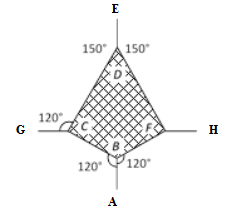# Second Law of Motion MCQs for NEET

The second law of motion states that The acceleration of an object as produced by a net force is directly proportional to the magnitude of the net force, in the same direction as the net force, and inversely proportional to the mass of the object.

1. A bullet of 5 gms travels with a velocity of 100 m/sec. It penetrates a wooden block up to 6 cm. What is the average force imposed on the block by the bullet?

1. 0
2. 417 N
3. 830 N
4. 830 N

2. The second law of motion gives the measure of which of the following?

1. Force
2. Acceleration
3. Momentum
4. Angular Momentum

3. What is the velocity of a body of mass 5 gm if a force of 100 dynes acts on the body for 10 seconds?

1. 2000 cm/sec
2. 200 cm/sec
3. 20 cm/sec
4. 2 cm/sec

4. An object will possess uniform motion as long as the

1. resultant force acting on it begins to decrease
2. resultant force on acting on it increases continuously
3. resultant force is right angles to its rotation
4. resultant force acting on it is zero

Answer: (d) resultant force acting on it is zero

5. What is the accelerating force on the Diwali rocket if it ejects 0.05 kg of gases per second at a velocity fo 400 m/s?

1. 20 N
2. 20 dynes
3. 1000 N
4. 22 dynes

6. A coin dropped in the lift takes time t1 to reach the floor when the lift is stationary and time t2 when the lift moves with a constant acceleration. Which of the following relation is true?

1. t1>t2
2. t2>t1
3. t1=t2
4. t1>>t2

7. A person standing in the elevator finds his weight to be lesser than his actual weight in which of the following cases?

1. When the elevator moves upward with constant acceleration
2. When the elevator moves downward with constant acceleration
3. When the elevator moves upward with constant velocity
4. When the elevator moves downward with constant velocity

Answer: (b) When the elevator moves downward with constant acceleration

8. A parachutist weighing w strikes the ground with his legs fixed and comes to rest with an upward acceleration of 3 g. What is the force exerted by him during landing?

1. w
2. 2w
3. 3w
4. 4w

9. A man stands on a weighing machine in the lift. When the lift is stationary his weight is recorded as 40 kg. If the lift accelerates upwards with an acceleration of 2 m/s2 what weight will the machine show?

1. 40 kg
2. 48 kg
3. 42 kg
4. 32 kg

10. Two trolleys of mass m and 3m are connected by a string. When they are compressed and released, they move off in opposite directions and come to rest after covering a distance of S1 and S2 respectively. Assuming the coefficient of friction to be constant, the ratio of S1: S2 is

1. 1:9
2. 1:3
3. 3:1
4. 9:1

11. What will happen if the body at rest is made to move in a straight line and obstructed by an opposite force?

1. The body slows down
2. The body changes direction
3. The body will continue to move in the same direction with the same speed
4. All of the above

Answer: (a) The body slows down

12. What is the apparent weight of the body travelling upwards with an acceleration of 2 m/s2 and has a mass of 10 kg?

1. 198 N
2. 140 N
3. 118 N
4. 164 N

13. On a body of 20 kg, a force of 10 N acts for 10 seconds. What is the change in its momentum?

1. 1000 kg.m/s
2. 200 kg.m/s
3. 100 kg.m/s
4. 5 kg.m/s

14. A machine gun fires a bullet of mass 40 g with a velocity of 1200 ms-1. The man holding it cam exert a maximum force of 144 N on the gun. How many bullets can he fire per second at the most?

1. One
2. Two
3. Three
4. Four

15. The figure shows a horizontal stretched net with a force of 10 N. The tension in sections BC and BF are1. 10 N, 10 N
2. 10 N, 11 N
3. 10 N, 6 N
4. Can’t calculate due to insufficient data

Answer: (a) 10 N, 10 N

Stay tuned to BYJU’S to get more updates on NEET.